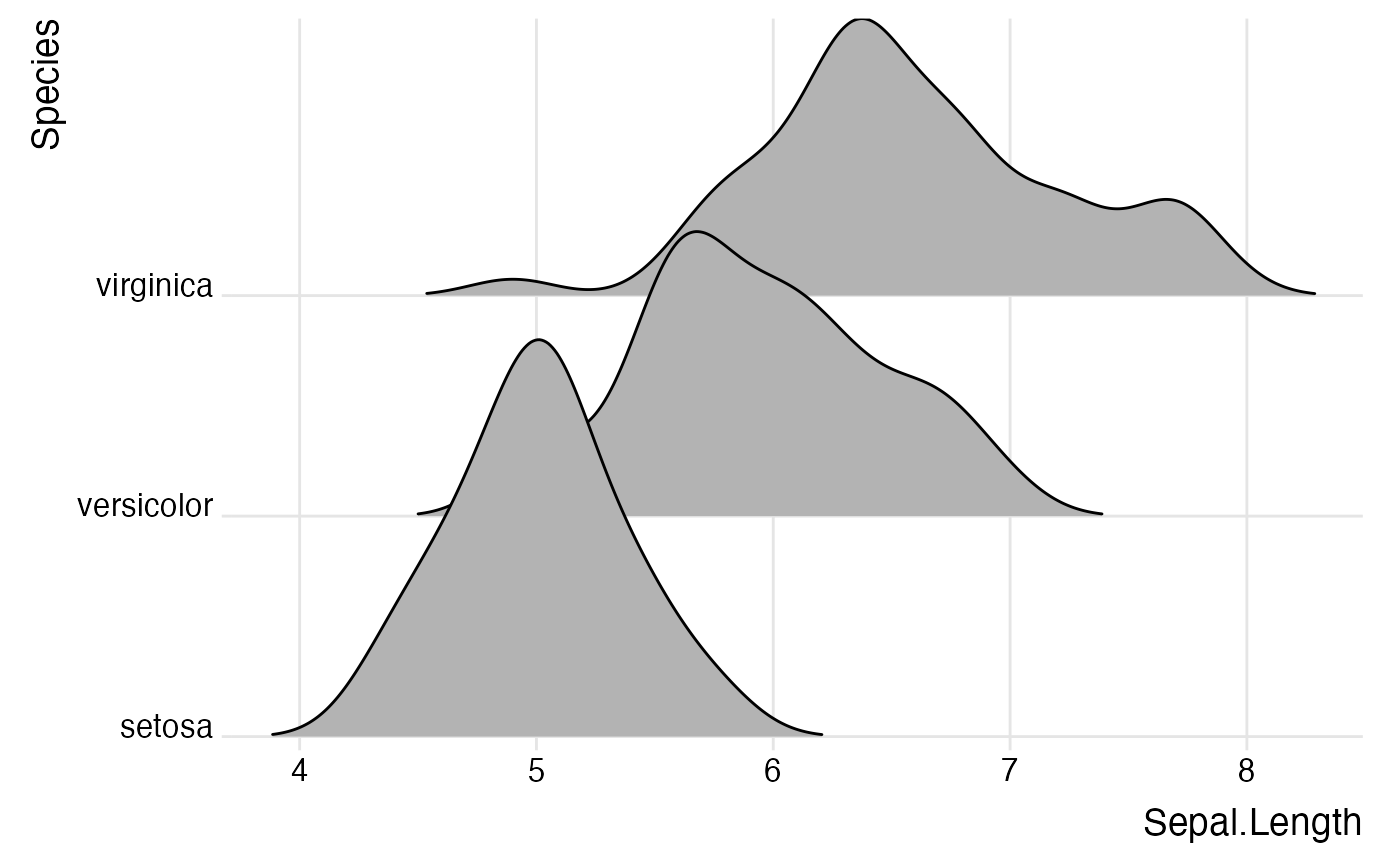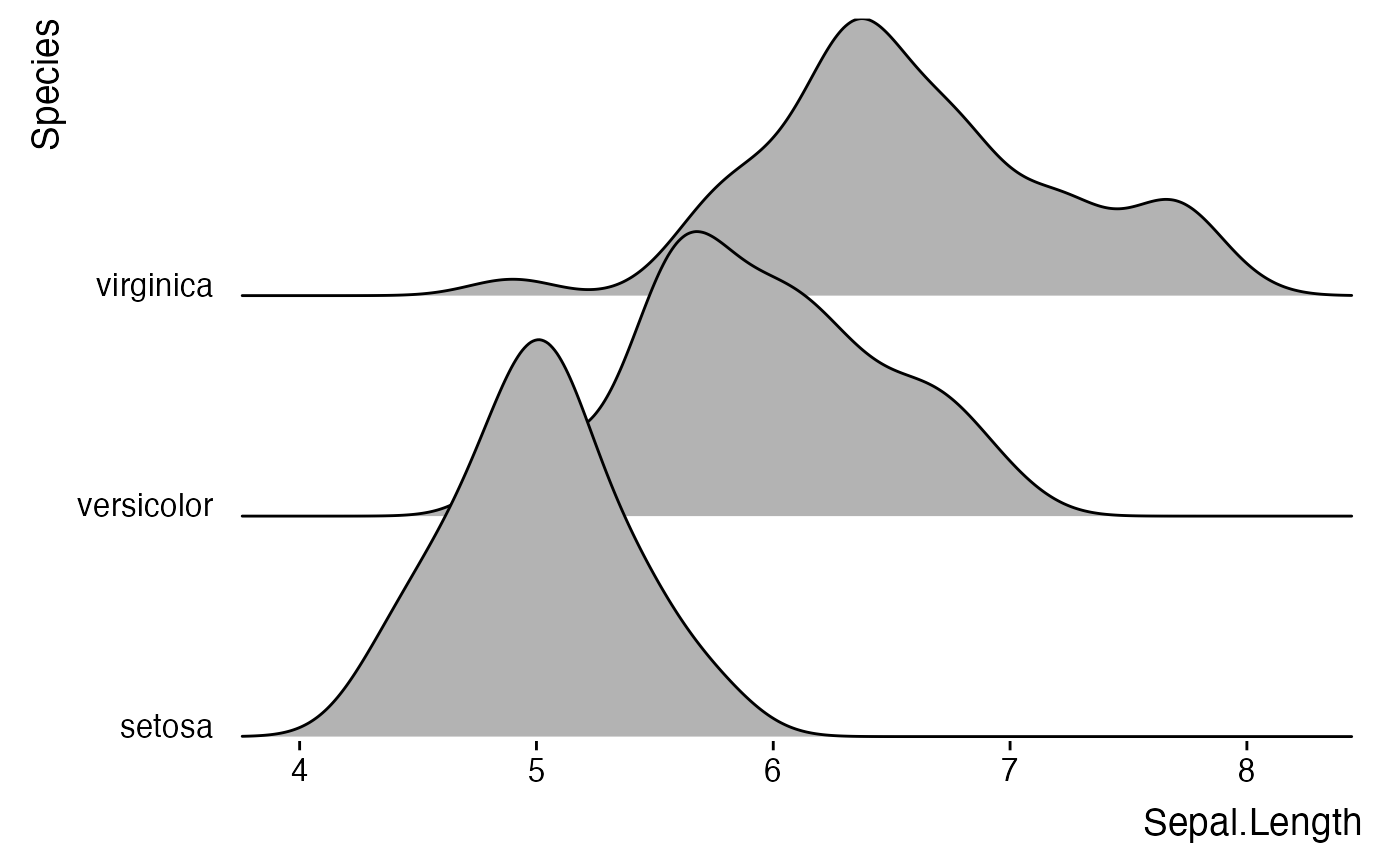This theme has some special modifications that make ridgeline plots look better, such as properly aligned y axis labels. It can draw plots with and without background grids (see examples).

theme_ridges(
font_size = 14,
font_family = "",
line_size = 0.5,
grid = TRUE,
center_axis_labels = FALSE
)

## Arguments

font_size

Overall font size. Default is 14.

font_family

Default font family.

line_size

Default line size.

grid

If TRUE (default), a background grid is drawn. If FALSE, background is left empty.

center_axis_labels

If TRUE, axis labels are drawn centered. If FALSE (default), axis lables are drawn right/top-aligned.

The theme.

## Examples

library(ggplot2)

# Example with background grid
ggplot(iris, aes(x = Sepal.Length, y = Species, group = Species)) +
geom_density_ridges(rel_min_height = 0.005) +
scale_y_discrete(expand = c(0.01, 0)) +
scale_x_continuous(expand = c(0.01, 0)) +
theme_ridges()
#> Picking joint bandwidth of 0.181# Example without background grid
ggplot(iris, aes(x = Sepal.Length, y = Species, group = Species)) +
geom_density_ridges() +
scale_y_discrete(expand = c(0.01, 0)) +
scale_x_continuous(expand = c(0.01, 0)) +
theme_ridges(grid = FALSE)
#> Picking joint bandwidth of 0.181